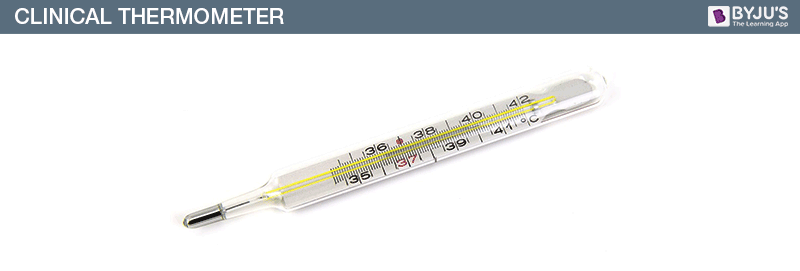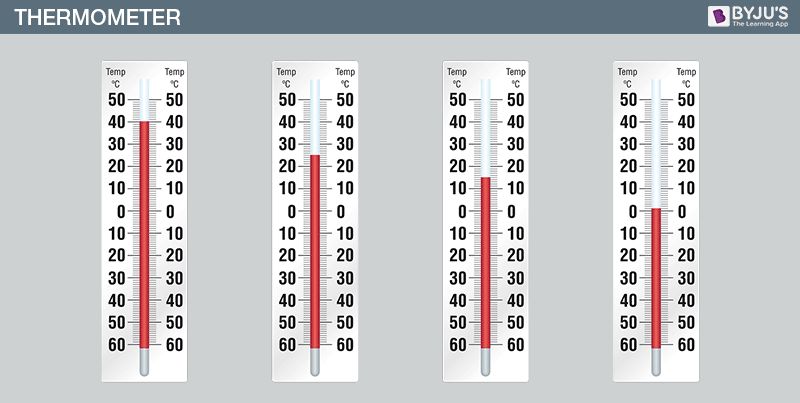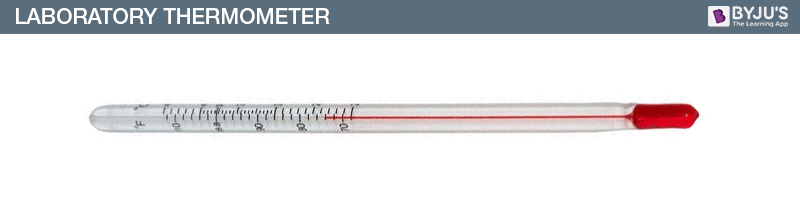# Thermometer: Clinical & Laboratory Thermometer

Thermometer (thermos: hot; metron: measure) is the universal instrument used to measure temperature. Temperature and heat are two words that often confuse people. For example, how do you explain the hotness of an object? What is the measure or basis for that hotness? The answer to that is temperature. Heat is a form of energy and its unit is Joules. On the other hand, Temperature is the measure of that heat. This means, if the heat is more, the temperature is more than well. But how do we measure temperature? We use a device called thermometer to measure the temperature of any object.

## Temperature & Thermometer

By definition, the temperature is the measurable extent of hotness or coldness. It is a mathematical representation of heat. There are different units to measure temperatures, like Celsius (˚C), Kelvin (K), and Fahrenheit (˚F).

There are different types of thermometers according to their needs. For example, there is one set of thermometers that are used to measure body temperature while another set of thermometers to measure boiling point and freezing point during experiments. Here, we will discuss the two types of thermometers, namely, clinical thermometer and laboratory thermometer.

### Properties of thermometric liquid

• The liquid that is used in the thermometer needs to have some properties, and they are listed below:
• The liquid used should be visible.
• The thermal expansion of the liquid must be uniform.
• The freezing point of the liquid used must be low.

## Clinical Thermometer

Clinical thermometers are meant for clinical purposes. It is developed for measuring the human body temperature. It is a long narrow glass tube with a bulb containing mercury at the end. The normal human body temperature is 37˚C; which can fluctuate between the ranges 35˚C to 42˚C. Hence, the clinical thermometers have the range 35˚C to 42˚C. The level of mercury tells our body temperature in ˚C. Since mercury is a toxic element, thus these thermometers have been replaced by digital thermometers nowadays.

Note: Clinical thermometers must be sterilized before use for the safe and clean check-up.### How to read a Thermometer?

• The thermometer is washed with water at a normal temperature.
• It is given a few jerks. We notice that the jerks bring the level of mercury down. We make sure that it falls below the 37⁰C mark or the 98⁰F mark, the normal human body temperature.
• The thermometer is then kept under the tongue or under the arms in order to get the reading on the thermometer.
• The thermometer is then held close to the eye as shown in figure 3 in order to see the correct reading.
• The temperature difference indicated between the two bigger marks is noted. Also, the number of divisions (shown by smaller marks) between these marks is noted. For e.g. the bigger mark reads 10 and the number of division is 5, then each division reads the value equal to 10/5 = 20• We note the value of the bigger mark that the mercury thread has crossed (which is 98 in the Fig.4 Example 1). Then the number of the division of the mercury thread has crossed is noted (3 in this case). Now, this value is multiplied by the value of one division (which is 0.2 in this case). Hence, the temperature reading is 98 + (3×2) = 98.6.

## Laboratory Thermometer

Since clinical thermometers can’t be used to measure temperature other than the human body, we need a special type of thermometers for other purposes. A laboratory thermometer, which is colloquially known as the lab thermometer, is used for measuring temperatures other than the human body temperature. It ranges from -10˚C to 110˚C. Laboratory thermometers are designed for lab purposes such as checking boiling point, freezing point, or temperature of other substances. You can use a laboratory thermometer for checking the temperature of a solvent but not a clinical one.Weather reporters also use maximum-minimum thermometers for measuring maximum and minimum temperatures of a place.

Watch the video below to understand the effect of temperature on matters surrounding us.1. Arkajit Roy

It is a good and intelligent app

2. Sahaj

Very nice for understanding the topics.

3. Sounak Sarkar

What an app this is, mind blowing. For the first time I am watching education with visualising.

4. Merinette Dupain Cheng

Nice explanation

5. Aloukik deep

Very good

6. Bhoomika m c

Explanation is nice